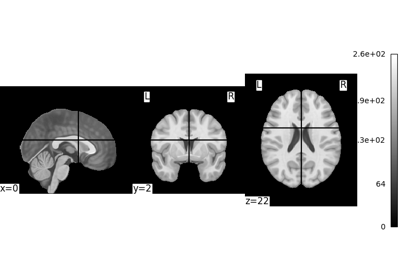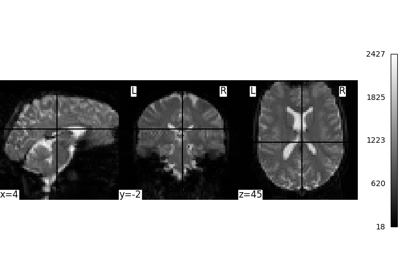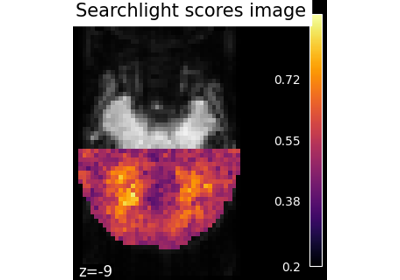Note

This page is a reference documentation. It only explains the function signature, and not how to use it. Please refer to the user guide for the big picture.

# nilearn.plotting.plot_img#

nilearn.plotting.plot_img(img, cut_coords=None, output_file=None, display_mode='ortho', figure=None, axes=None, title=None, threshold=None, annotate=True, draw_cross=True, black_bg=False, colorbar=False, cbar_tick_format='%.2g', resampling_interpolation='continuous', bg_img=None, vmin=None, vmax=None, radiological=False, **kwargs)[source]#

Plot cuts of a given image.

By default Frontal, Axial, and Lateral.

Parameters:
imgNiimg-like object
cut_coordsNone, a `tuple` of `float`, or `int`, optional

The MNI coordinates of the point where the cut is performed.

• If display_mode is ‘ortho’ or ‘tiled’, this should be a 3-tuple: (x, y, z)

• For display_mode == “x”, “y”, or “z”, then these are the coordinates of each cut in the corresponding direction.

• If None is given, the cuts are calculated automatically.

• If display_mode is ‘mosaic’, and the number of cuts is the same for all directions, cut_coords can be specified as an integer. It can also be a length 3 tuple specifying the number of cuts for every direction if these are different.

Note

If display_mode is “x”, “y” or “z”, cut_coords can be an integer, in which case it specifies the number of cuts to perform.

output_file`str`, or None, optional

The name of an image file to export the plot to. Valid extensions are .png, .pdf, .svg. If output_file is not None, the plot is saved to a file, and the display is closed.

display_mode{“ortho”, “tiled”, “mosaic”, “x”, “y”, “z”, “yx”, “xz”, “yz”}, default=”ortho”

Choose the direction of the cuts:

• “x”: sagittal

• “y”: coronal

• “z”: axial

• “ortho”: three cuts are performed in orthogonal directions

• “tiled”: three cuts are performed and arranged in a 2x2 grid

• “mosaic”: three cuts are performed along multiple rows and columns

figure`int`, or `matplotlib.figure.Figure`, or None, optional

Matplotlib figure used or its number. If None is given, a new figure is created.

axes`matplotlib.axes.Axes`, or 4 tupleof `float`: (xmin, ymin, width, height), default=None

The axes, or the coordinates, in matplotlib figure space, of the axes used to display the plot. If None, the complete figure is used.

title`str`, or None, default=None

The title displayed on the figure.

thresholda number, None, or ‘auto’, optional

If None is given, the image is not thresholded. If a number is given, it is used to threshold the image: values below the threshold (in absolute value) are plotted as transparent. If “auto” is given, the threshold is determined magically by analysis of the image.

annotate`bool`, default=True

If annotate is True, positions and left/right annotation are added to the plot.

decimalsinteger, optional

Number of decimal places on slice position annotation. If False (default), the slice position is integer without decimal point.

draw_cross`bool`, default=True

If draw_cross is True, a cross is drawn on the plot to indicate the cut position.

black_bg`bool`, or “auto”, optional

If True, the background of the image is set to be black. If you wish to save figures with a black background, you will need to pass facecolor=”k”, edgecolor=”k” to `matplotlib.pyplot.savefig`. Default=False.

colorbar`bool`, optional

If True, display a colorbar on the right of the plots. Default=False.

cbar_tick_format: str, optional

Controls how to format the tick labels of the colorbar. Ex: use “%i” to display as integers. Default is ‘%.2g’ for scientific notation.

resampling_interpolation`str`, optional

Interpolation to use when resampling the image to the destination space. Can be:

• “continuous”: use 3rd-order spline interpolation

• “nearest”: use nearest-neighbor mapping.

Note

“nearest” is faster but can be noisier in some cases.

Default=’continuous’.

bg_imgNiimg-like object, optional

See Input and output: neuroimaging data representation. The background image to plot on top of. If nothing is specified, no background image is plotted. Default=None.

vmin`float`, optional

Lower bound of the colormap. If None, the min of the image is used. Passed to `matplotlib.pyplot.imshow`.

vmax`float`, optional

Upper bound of the colormap. If None, the max of the image is used. Passed to `matplotlib.pyplot.imshow`.

radiological`bool`, default=False

Invert x axis and R L labels to plot sections as a radiological view. If False (default), the left hemisphere is on the left of a coronal image. If True, left hemisphere is on the right.

kwargsextra keyword arguments, optional

Extra keyword arguments passed to matplotlib.pyplot.imshow.

## Examples using `nilearn.plotting.plot_img`#Basic nilearn example: manipulating and looking at data

Basic nilearn example: manipulating and looking at dataIntro to GLM Analysis: a single-session, single-subject fMRI dataset

Intro to GLM Analysis: a single-session, single-subject fMRI datasetSearchlight analysis of face vs house recognition

Searchlight analysis of face vs house recognition